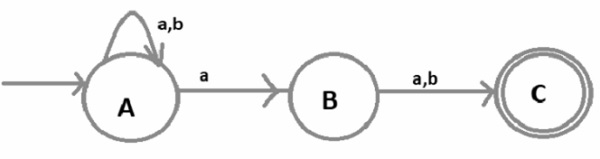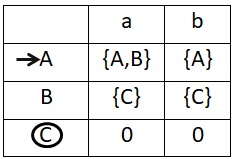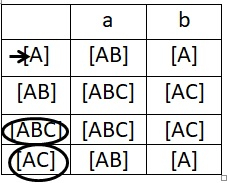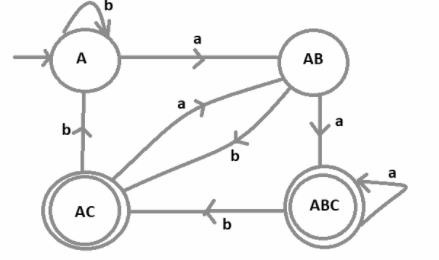# Construct DFA of a string in which the second symbol from RHS is ‘a’

A Deterministic Finite automaton (DFA) is a 5-tuples

M=(Q, Σ, δ,q0,F)

Where,

• Q : Finite set called states.
• Σ : Finite set called alphabets.
• δ : Q × Σ → Q is the transition function.
• q0 ϵ Q is the start or initial state.
• F : Final or accept state.

## Problem

Design a finite automaton where the second symbol from the right hand side is ‘a’.

## Solution

The language for a given string over an alphabet {a,b} is −

L={aa,abaa,abbab,aaabab,………}

## Example

Input − aaba

Output − Not accepted

Because the second letter from right hand side is not a

Input − aabbab

Output − Accepted

It is complicated to directly construct the DFA.

So, first try to design the non-deterministic finite automata (NFA) and then convert it to the deterministic finite automata (DFA).

Now construct the NFA of the language containing all the strings where the 2nd symbol from the RHS is “a” is given below −Here,

• A is the initial state.
• B is the intermediate state and
• C is the final state.

Construct NFA transition table. With the help of a transition table, it is easy to convert the DFA transition table from there to a DFA transition diagram.

The NFA transition table is as follows −Now convert this NFA transition table to DFA transition table.

We can do this by using the subset configuration on the state transition table of NFA.

The DFA transition table is as follows −Now, it is comparatively easy to draw the DFA with the help of its transition table.

In this DFA, there are four different states A, AB, ABC and AC, where ABC and AC are the final states because c is the final state in NFA, wherever C is present that state becomes the final state and A is the initial state of the DFA.